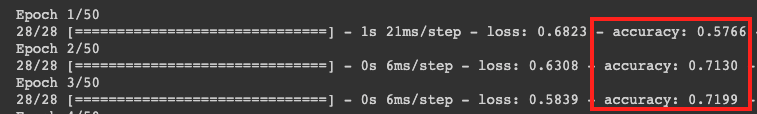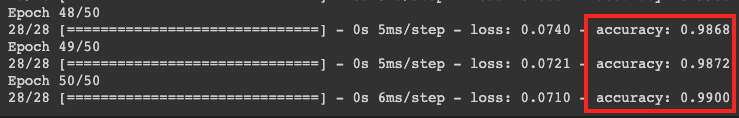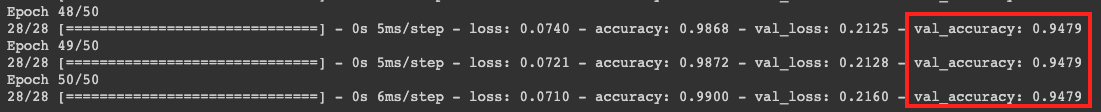# Train a comment-spam detection model with TensorFlow Lite Model Maker

## 1. Before you begin

In this codelab, you review code created with TensorFlow and TensorFlow Lite Model Maker to create a model with a dataset based on comment spam. The original data is available on Kaggle. It's been gathered into a single CSV file, and cleaned up by removing broken text, markup, repeated words and more. This will make it easier to focus on the model instead of the text.

The code that you review is supplied here, but it's highly recommended that you follow along with the code in Colaboratory.

## What you'll learn

• How to install TensorFlow Lite Model Maker with Colab.
• How to use a data loader.
• How to build the model.

## 2. Install TensorFlow Lite Model Maker

• Open the Colab. The first cell in the notebook will install TensorFLow Lite Model Maker for you:
``````!pip install -q tflite-model-maker
``````

Once it has completed, move on to the next cell.

## 3. Import the code

The next cell has a number of imports that the code in the notebook will need to use:

``````import numpy as np
import os
from tflite_model_maker import configs
from tflite_model_maker import ExportFormat
from tflite_model_maker import model_spec
from tflite_model_maker import text_classifier

import tensorflow as tf
assert tf.__version__.startswith('2')
tf.get_logger().setLevel('ERROR')
``````

This will also check to see if you ran TensorFlow 2.x, which is a requirement to use Model Maker.

Next you'll download the data from the Colab server to your device, and set the `data_file` variable to point at the local file:

``````data_file = tf.keras.utils.get_file(fname='comment-spam.csv',
extract=False)
``````

Model Maker can train models from simple CSV files like this one. You only need to specify which columns hold the text and which hold the labels, which you see how to do later in this codelab.

## 5. Pre-learned embeddings

Generally, when you use Model Maker, you don't build models from scratch. You use existing models that you customize to your needs.

Language models, like this one, involve the use of pre-learned embeddings. The idea behind an embedding is that words are converted into numbers with each word in your overall corpus given a number. An embedding is a vector that's used to determine the sentiment of that word by establishing a "direction" for the word. For example, words that are used frequently in comment-spam messages have their vectors point in a similar direction and words that aren't point have their vectors point in the opposite direction.

When you use pre-learned embeddings, you get to start with a corpus, or collection, of words that have already had sentiment learned from a large body of text, so you get to a solution much faster than when you start from zero.

Model Maker provides several pre-learned embeddings that you can use, but the simplest and quickest one to begin with is `average_word_vec` option.

Here's the code for it:

``````spec = model_spec.get('average_word_vec')
spec.num_words = 2000
spec.seq_len = 20
spec.wordvec_dim = 7
``````

## The `num_words` parameter

You also specify the number of words that you want your model to use.

You might think "the more the better," but there's generally a right number based on the frequency that each word is used. If you use every word in the entire corpus, the model could try to learn and establish the direction of words that are only used once. In any text corpus, many words are only used once or twice, so their inclusion in your model isn't worthwhile because they have a negligible impact on the overall sentiment.

You can use the `num_words` parameter to tune your model based on the number of words that you want. A smaller number might provide a smaller and quicker model, but it could be less accurate because it recognizes fewer words. On the other hand, a larger number might provide a larger and slower model. It's important to find the sweet spot!

## The `wordvec_dim` parameter

The `wordved_dim` parameter is the number of dimensions that you want to use for the vector for each word. The rule of thumb determined from research is that it's the fourth root of the number of words. For example, if you use 2,000 words, 7 is a good starting point. If you change the number of words that you use, you can also change this.

## The `seq_len` parameter

Models are generally very rigid when it comes to input values. For a language model, this means that the language model can classify sentences of a particular static length. That's determined by the `seq_len` parameter or sequence length.

When you convert words into numbers or tokens, a sentence then becomes a sequence of these tokens. In this case, your model is trained to classify and recognize sentences with 20 tokens. If the sentence is longer than this, it's truncated. If it's shorter, it's padded. You can see a dedicated `<PAD>` token in the corpus that's used for this.

## 6. Use a data loader

Earlier you downloaded the CSV file. Now it's time to use a data loader to turn this into training data that the model can recognize:

``````data = DataLoader.from_csv(
filename=data_file,
text_column='commenttext',
label_column='spam',
model_spec=spec,
delimiter=',',
shuffle=True,
is_training=True)

train_data, test_data = data.split(0.9)
``````

If you open the CSV file in an editor, you'll see that each line just has two values, and these are described with text in the first line of the file. Typically, each entry is then deemed to be a column.

You'll see that the descriptor for the first column is `commenttext`, and that the first entry on each line is the text of the comment. Similarly, the descriptor for the second column is `spam`, and you'll see that the second entry on each line is `True` or `False,` to denote if that text is considered comment spam or not. The other properties set the `model_spec` variable that you created earlier, along with a delimiter character, which in this case is a comma as the file is comma separated. You will use this data for training the model, so `is_Training` is set to `True`.

You will want to hold back a portion of the data for testing the model. Split the data, with 90% of it for training, and the other 10% for testing/evaluation. Because we're doing this we want to make sure that the testing data is chosen at random, and isn't the ‘bottom' 10% of the dataset, so you use `shuffle=True` when loading the data to randomize it.

## 7. Build the model

The next cell is simply to build the model, and it's a single line of code:

``````# Build the model
model = text_classifier.create(train_data, model_spec=spec, epochs=50,
validation_data=test_data)
``````

This code creates a text-classifier model with Model Maker and you specify the training data that you want to use as set up in fourth step), the model specification as set up in the fourth step, and a number of epochs, which is 50 in this case.

The basic principle of ML is that it's a form of pattern matching. Initially, it loads the pre-trained weights for the words and attempts to group them together with a prediction of which ones, when grouped together, indicate spam and which ones don't. The first time around, it's likely to be evenly split because the model is only getting started.It will then measure the results of this epoch of training and run optimization code to tweak its prediction, then try again. This is an epoch. So, by specifying epochs=50, it will go through that "loop" 50 times.By the time you reach the 50th epoch, the model reports a much higher level of accuracy. In this case, it shows `99%`!

The validation accuracy figures are typically a bit lower than the training accuracy because they're an indication of how the model classifies data that it hasn't previously seen. It uses the 10% test data that you set aside earlier.## 8. Export the model

1. Run this cell to specify a directory and export the model:
``````model.export(export_dire='/mm_spam_savedmodel', export_format=[ExportFormat.LABEL, ExportFormat.VOCAB, ExportFormat.SAVED_MODEL])
``````
1. Compress the entire folder of `/mm_spam_savedmodel` and down the generated `mm_spam_savedmodel.zip` file, which you need in the next codelab.
``````# Rename the SavedModel subfolder to a version number
!mv /mm_spam_savedmodel/saved_model /mm_spam_savedmodel/123
!zip -r mm_spam_savedmodel.zip /mm_spam_savedmodel/
``````

## 9. Congratulations

This codelab took you through Python code to build and export your model. Now you have a SavedModel plus the labels and vocabulary at the end of it. In the next codelab, you see how to use this model so that you can begin to classify spam comments.